﻿ Geometric Figures, Trefoil Icon. Elements Of Geometric Figures Illustration Icon. Signs And Symbols Can Be Used For Web, Logo, Stock Vector - Illustration of illustration, abstract: 145501239

# Geometric figures, trefoil icon. Elements of geometric figures illustration icon. Signs and symbols can be used for web, logo, .

## Geometric figures, trefoil icon. Elements of geometric figures illustration icon. Signs and symbols can be used for web, logo, mobile app, UI, UX on white background

More similar stock illustrations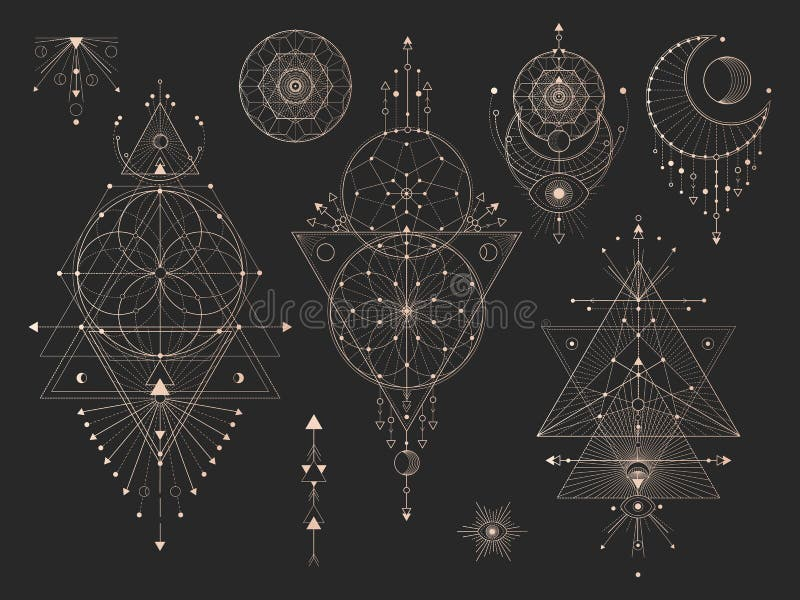Vector set of Sacred geometric symbols with moon, eye, arrows, dreamcatcher and figures on black background. Gold abstract mystic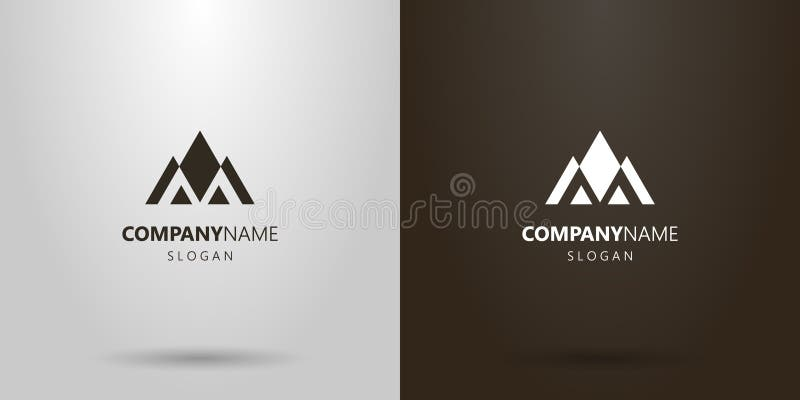Simple vector geometric logo of a mountain landscape of triangular figures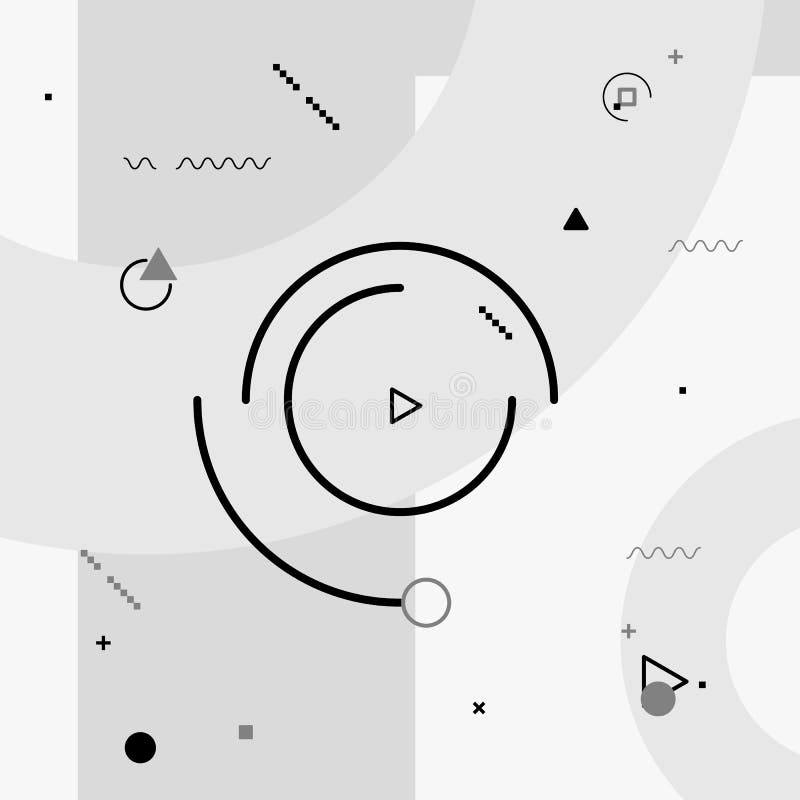Motion graphics elements. Black and white composition. Vector illustration background. Geometric figures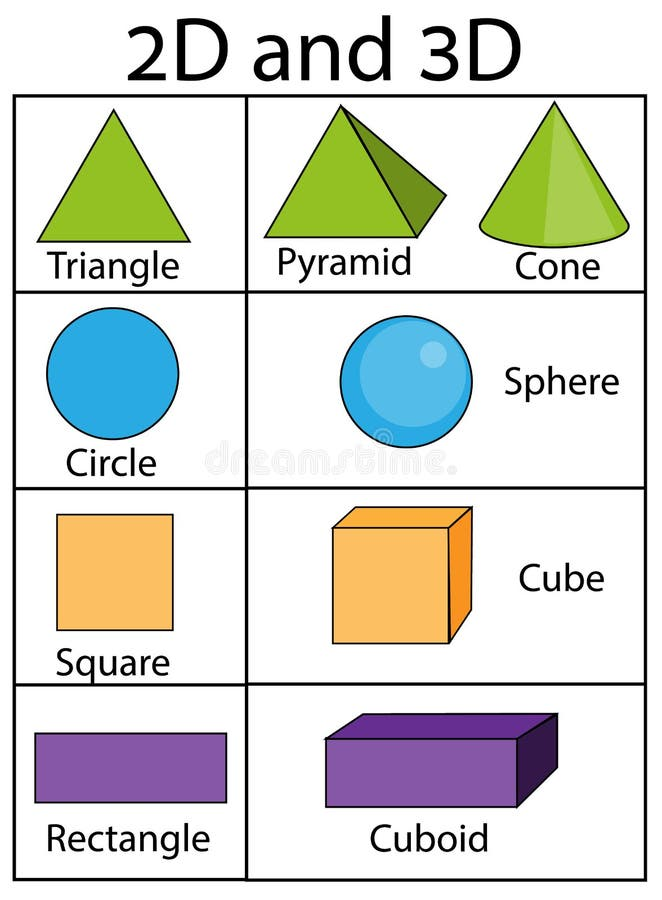2D and 3D geometric shapes and figures. Educational infographics for kids. Children visual aids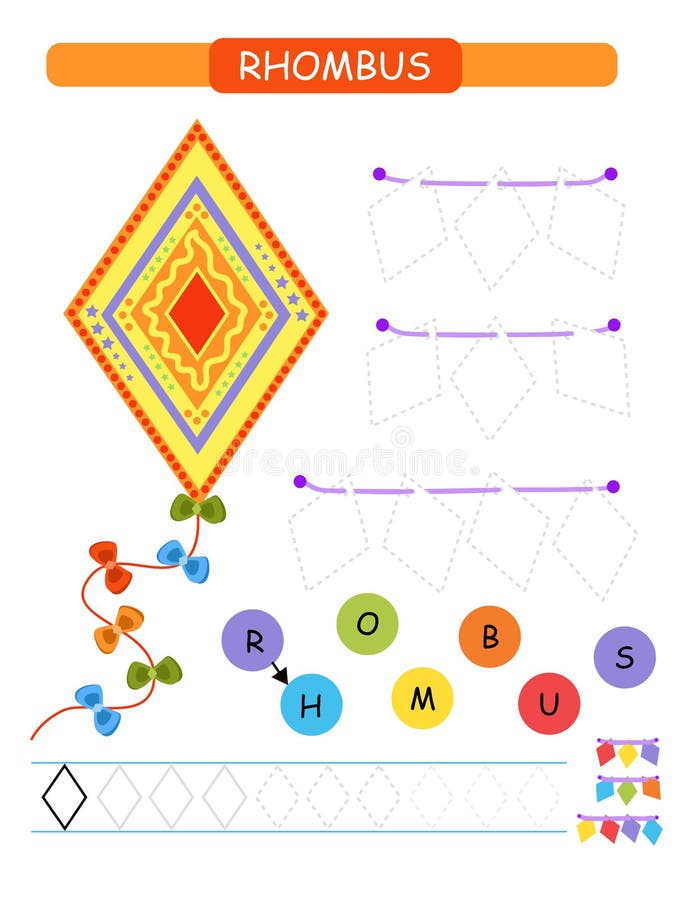Learn shapes and geometric figures for preschool and kindergarten printable worksheet. Cartoon vector illustration -rhombus.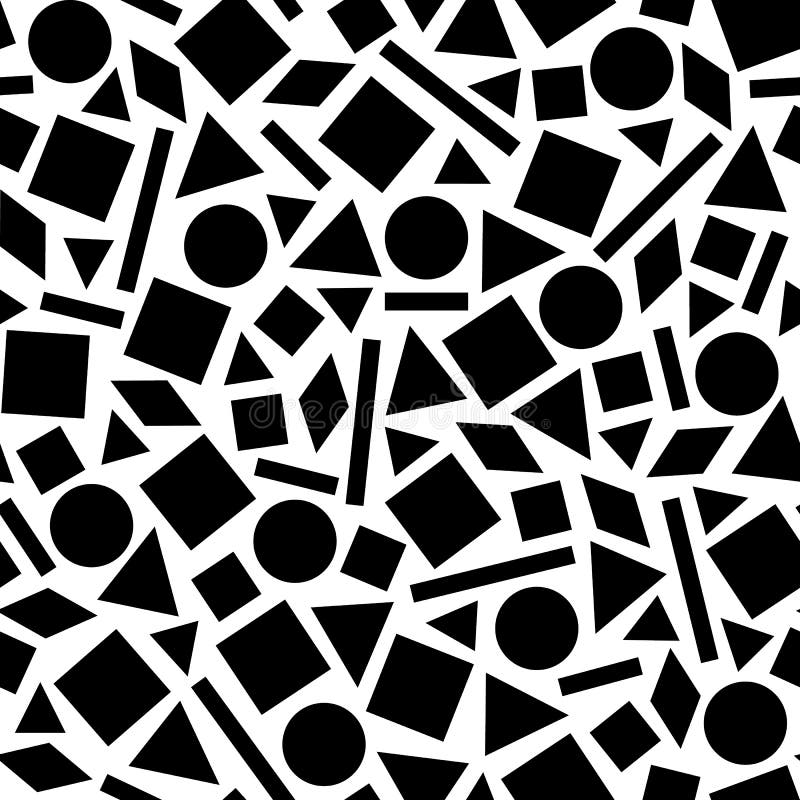Geometric pattern of the figures.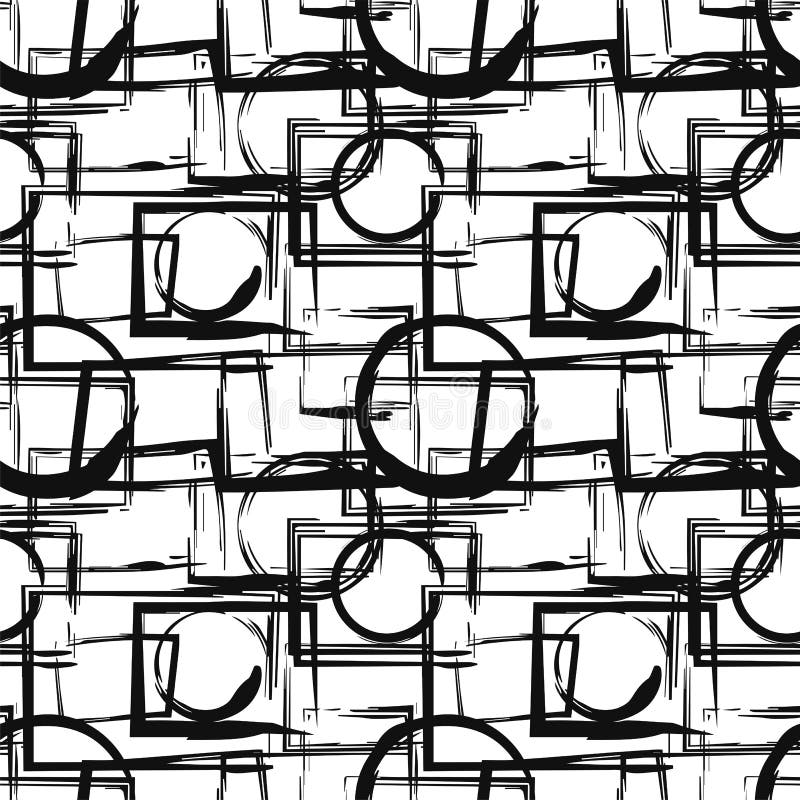Seamless pattern with abstract black geometric figures in grunge style. Vector design elements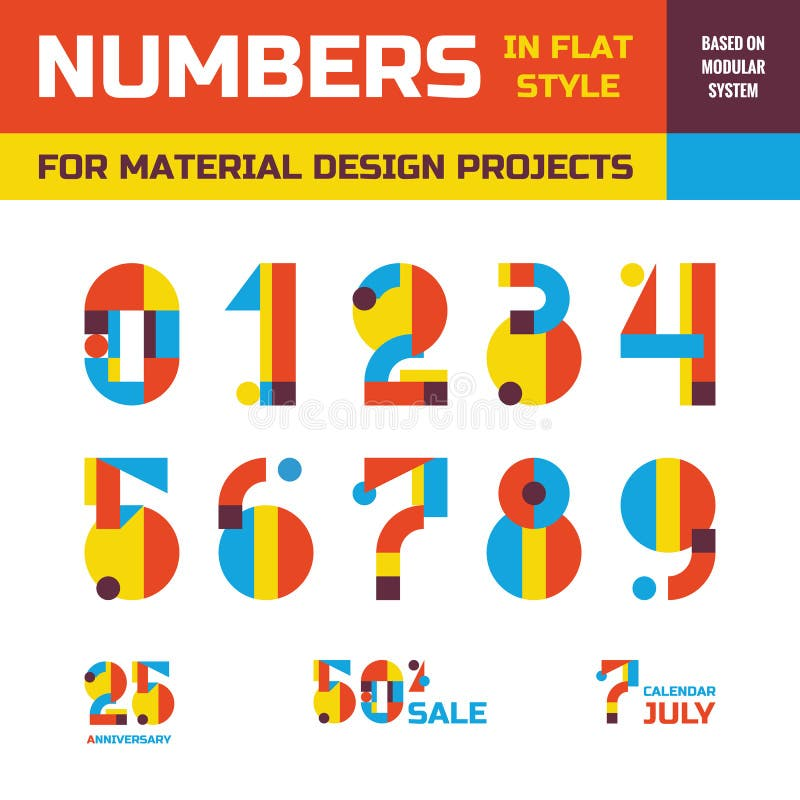Abstract vector numbers in flat style design for material design creative projects. Geometric numbers symbols. Decorative figures.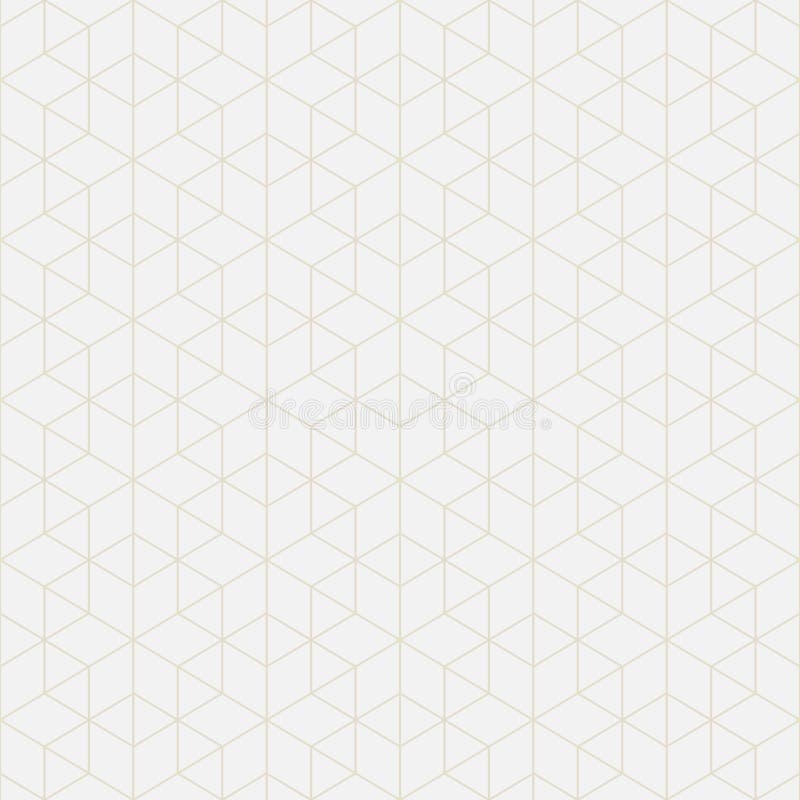Mathematical figures. Abstract geometric background.
More stock photos from Fidan Babayeva's portfolio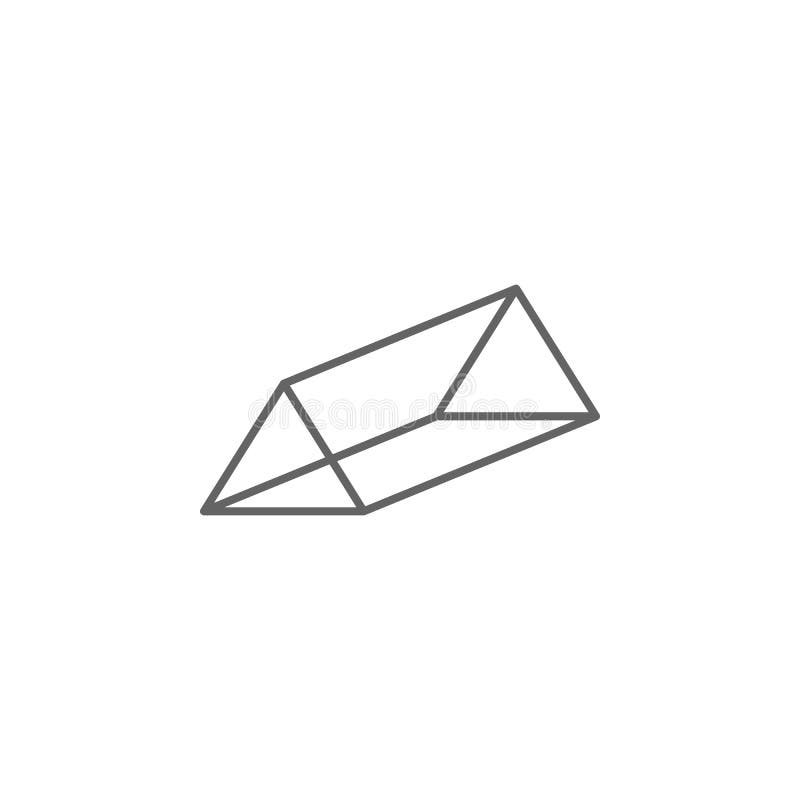Geometric figures, triangular prism outline icon. Elements of geometric figures illustration icon. Signs and symbols can be used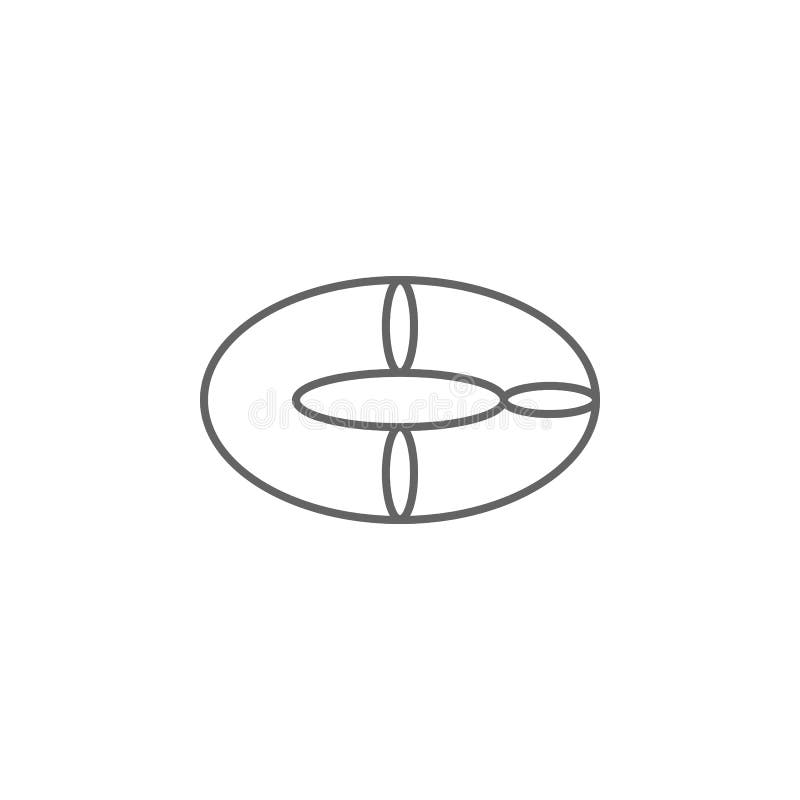Geometric figures, torus outline icon. Elements of geometric figures illustration icon. Signs and symbols can be used for web,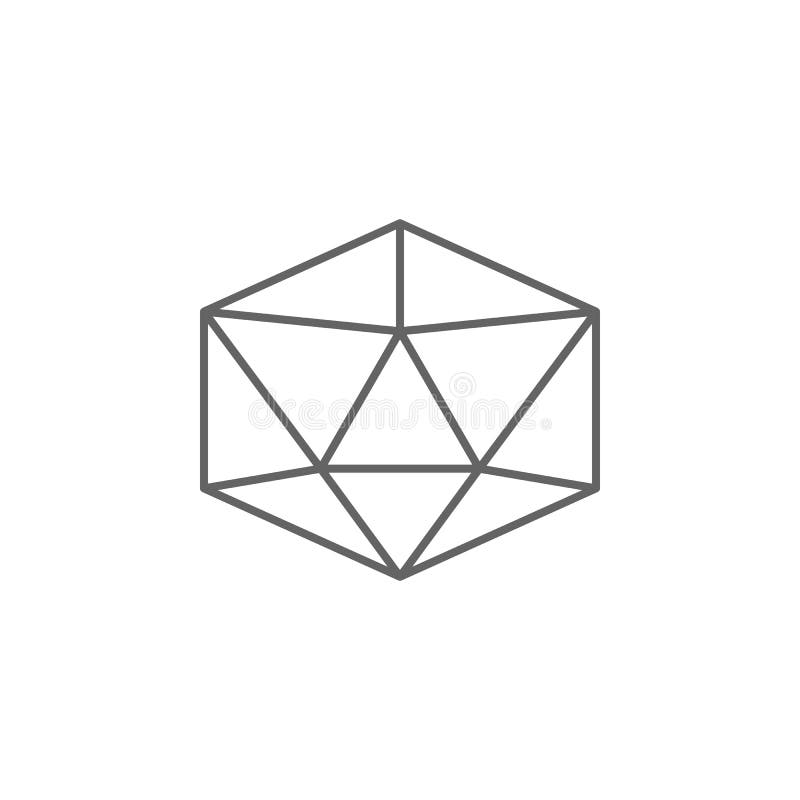Geometric figures, icosahedron outline icon. Elements of geometric figures illustration icon. Signs and symbols can be used for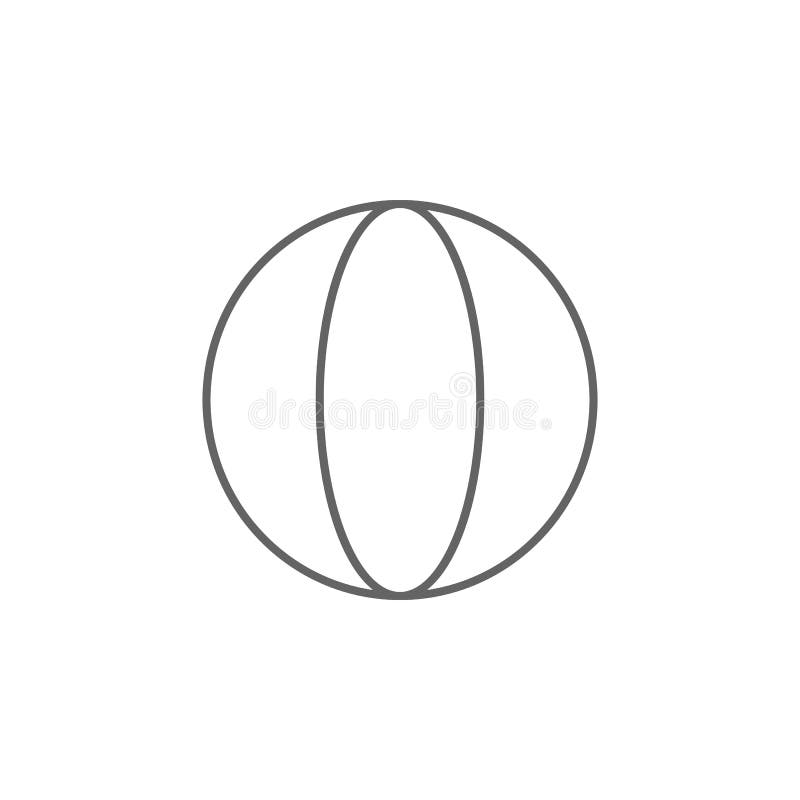Geometric figures, sphere outline icon. Elements of geometric figures illustration icon. Signs and symbols can be used for web,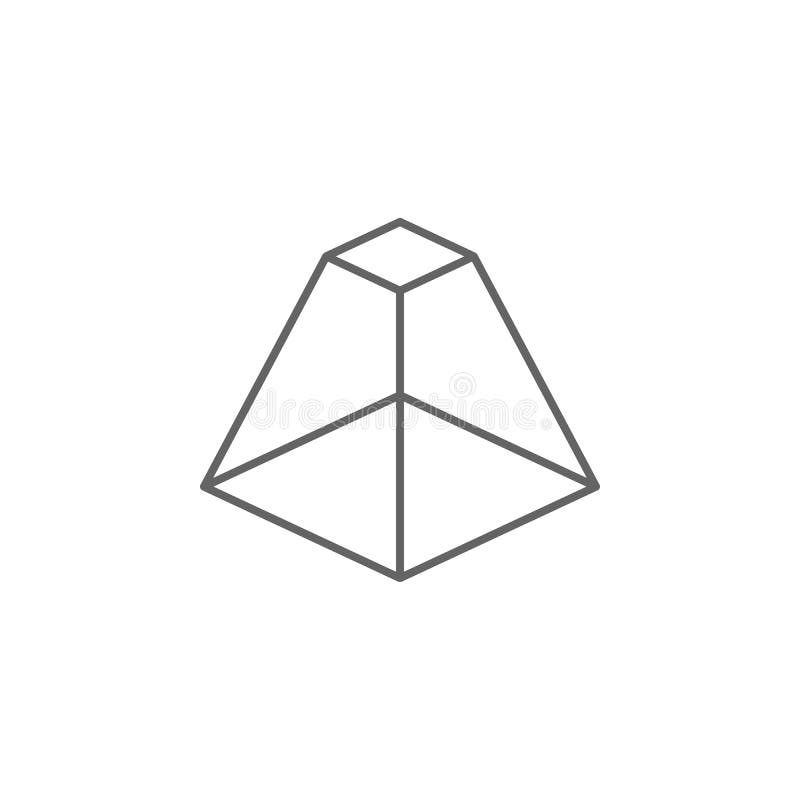Geometric figures, pyramid with flat top outline icon. Elements of geometric figures illustration icon. Signs and symbols can be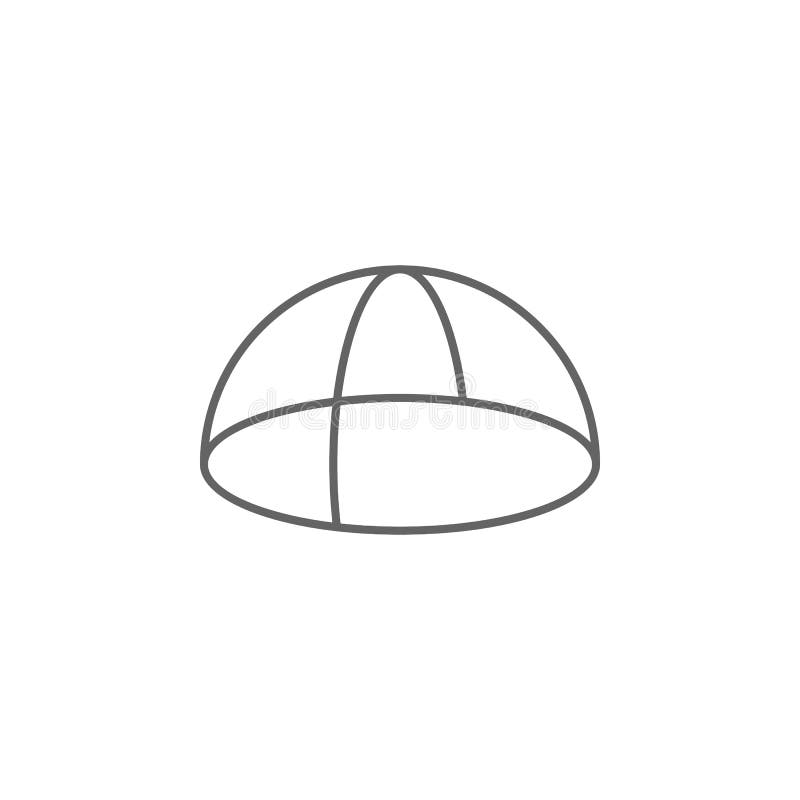Geometric figures, hemisphere outline icon. Elements of geometric figures illustration icon. Signs and symbols can be used for web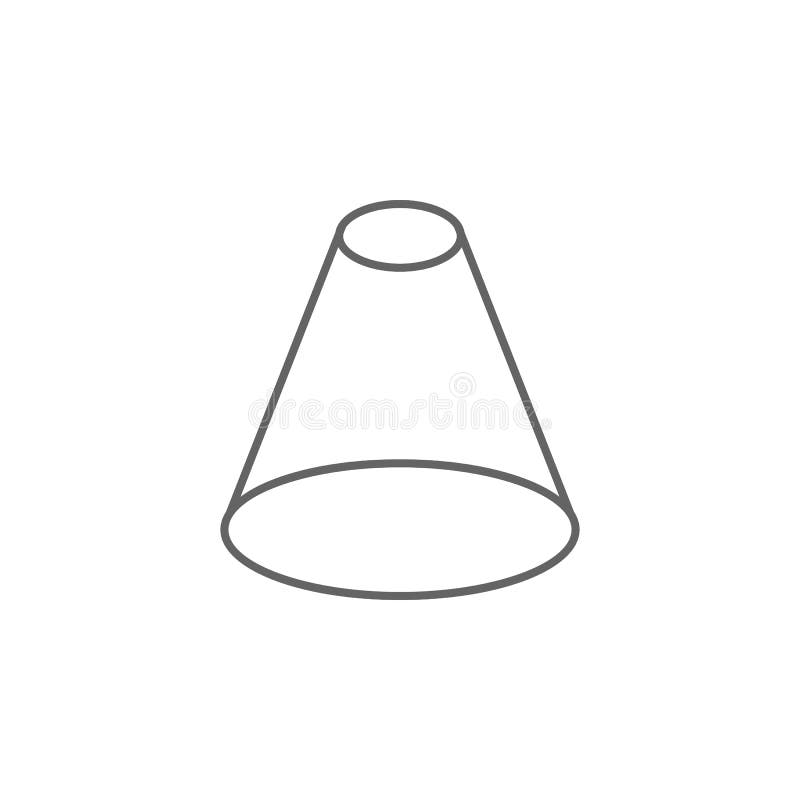Geometric figures, cone with flat top outline icon. Elements of geometric figures illustration icon. Signs and symbols can be used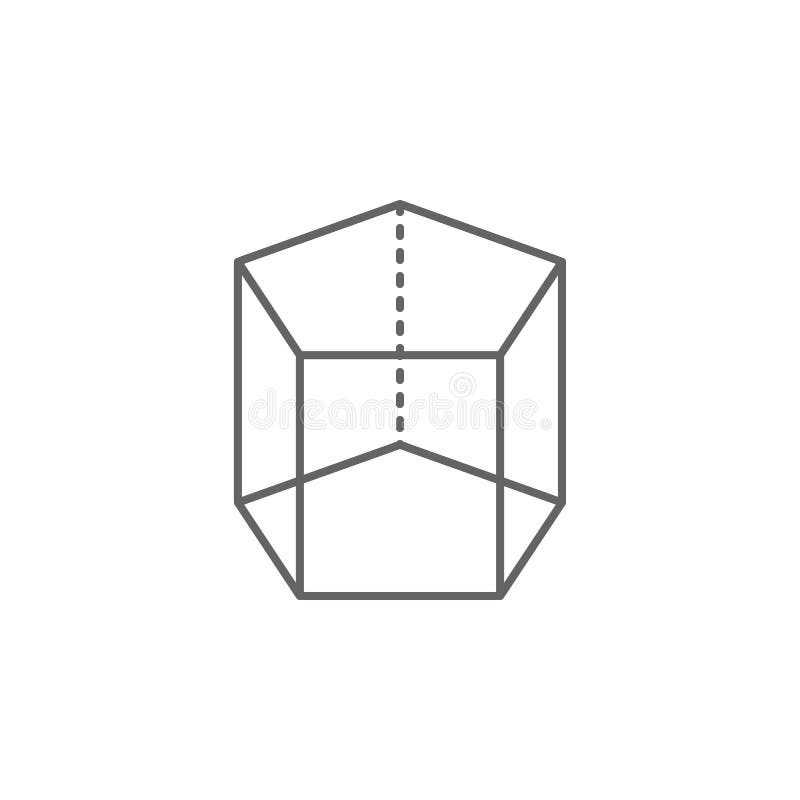Geometric figures, pentagonal prism outline icon. Elements of geometric figures illustration icon. Signs and symbols can be used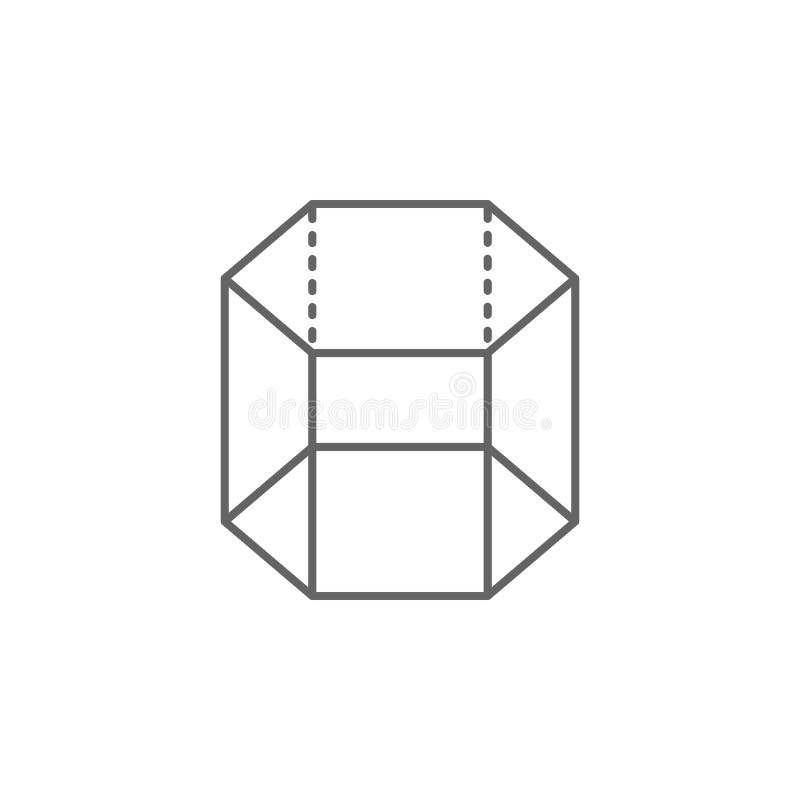Geometric figures, hexagonal prism outline icon. Elements of geometric figures illustration icon. Signs and symbols can be used
Related categories
Extended licenses